# Survival analysis

David Springate
March 2014

### Classically, the analysis of time to death.

But can be used anywhere you want to know what factors affect the time for an event to occur:

• Germination timing
• Arrival of a migrant or parasite
• Dispersal of seeds or offspring
• Failure time in mechanical systems
• Response to stimulus

## Right censoring:

Where the date of death is unknown but is after some known date

e.g.

• Date of death is after the end of the study
• Subject is removed from the study (patient withdraws, animal escapes, plant gets eaten etc.)

Survival analysis can account for this kind of censoring

## Left censoring:

Occurs when a subject's survival time is incomplete on the left side of the follow-up period

• e.g. Following up a patient after being tested for an infection, we don't know the exact time of exposure
• Less common
• Survival analysis can account for this (see ref 4)

### The Survival Function (Survival curve)

$S(t)=Pr(T>t)$

The Survival function ($$S$$) is the probability that the time of death ($$T$$) is greater than some specified time ($$t$$)

It is composed of:

• The underlying Hazard function (How the risk of death per unit time changes over time at baseline covariates)
• The effect parameters (How the hazard varies in response to the covariates)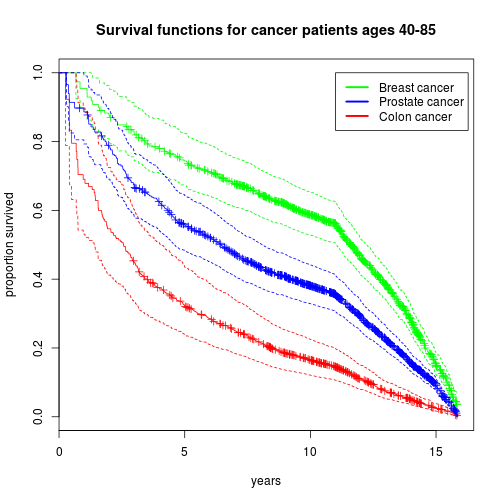### The Cox Proportional-Hazards Model

The most common model used to determine the effects of covariates on survival

$h_i(t)=h_0(t)exp(\beta_{1}x_{i1} + \beta_{2}x_{ik} + ... + \beta_{2}x_{ik} )$

It is a semi-parametric model:

• The baseline hazard function is unspecified
• The effects of the covariates are multiplicative
• Doesn't make arbitrary assumptions about the shape/form of the baseline hazard function

### The Proportional Hazards Assumption

• Covariates multiply the hazard by some constant
• e.g. a drug may halve a subjects risk of death at any time $$t$$
• The effect is the same at any time point

## Violating the PH assumption can seriously invalidate your model!

### Survival analysis in R

Uses the survival package.

The response variable is a Surv object taking in

• start time (after study start)
• stop time (after study start)
• whether or not an event occurred

Otherwise, the model is specified in the same way as for a standard regression, using the coxph function

Toolkit

Surv(): Define a survival object

coxph(): Run a cox PH regression

survfit(): Fit a survival curve to a model or formula

### Effect of Beta-blockers on colon cancer survival rates

From the electronic medical records of 13 million UK patients, I identified:

• Patients aged 40-85 with a first diagnosis of colon cancer between 1997 and 2006
• Classified according to exposure to $$\beta$$-blockers in the 1 year period prior to diagnosis
• Patients with heart disease, stroke, asthma, diabetes, kidney disease etc. were excluded
• controlling for sex, age, year of diagnosis, numer of drugs being taken, smoking status, level of social deprivation

1104 patients not on $$\beta$$-blockers - CONTROL
695 patients on $$\beta$$-blockers - TREATMENT

### Effect of Beta-blockers on colon cancer survival rates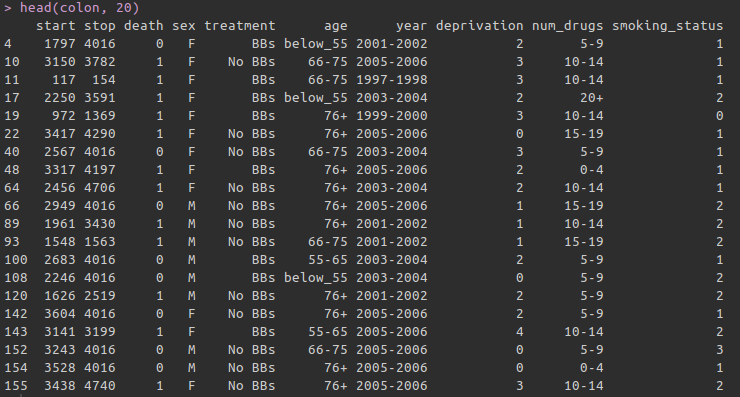### Setting up the response variable

• Start time: time (days) of diagnosis date after the study start date
• Stop time: time to whichever comes first from
• Study end
• patient dies
• patient leaves practice
• event: binary does the patient die?

# Create the response variable:
S <- Surv(
time = colon$start, time2 = colon$stop,
event = colon$death)  This includes the right censoring information with the survival time ### Building the model model <- coxph(S ~ sex + treatment + age + year + deprivation + num_drugs + smoking_status, data = colon)  • Use the coxph function • The new response variable goes on the LHS • Otherwise, it uses the same syntax as lm(), glm() etc. ### Model results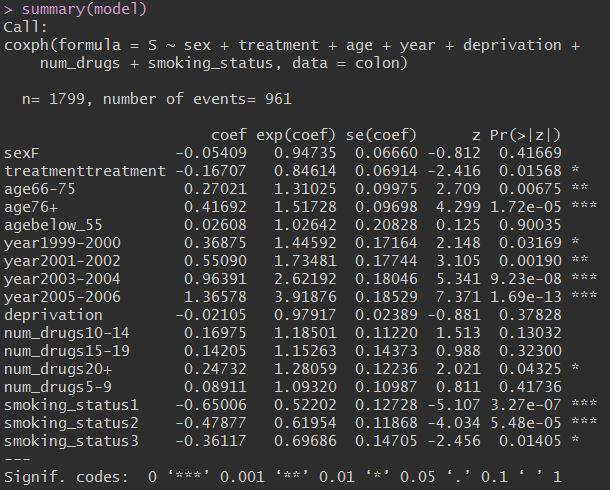The exp(coef) variable is the Hazard ratio: The multiplicative effect of the covariate on the Hazard function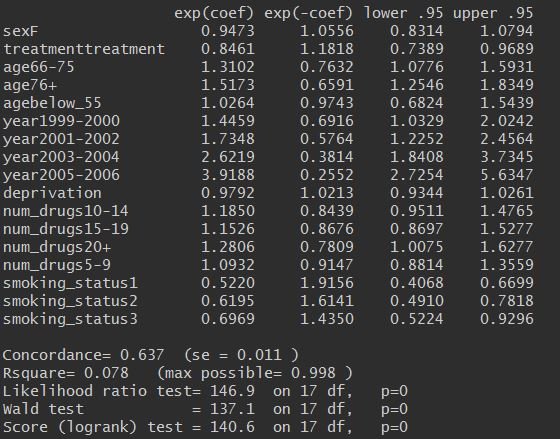• HR = 1: No effect • HR < 1: Reduction in the hazard • HR > 1: Increase in Hazard ### Model results # Plot the baseline survival function plot(survfit(model), xscale = 365.25, xlab = "Years after diagnosis", ylab = "Proportion survived", main = "Baseline Hazard Curve")### Plotting the effects First, build a dataframe for the effect levels you want to look at Other covariates are held at their lowest level (or means if they are continuous) treat <- with(colon, data.frame( treatment = levels(treatment), age = rep(levels(age), 2), sex = rep(levels(sex), 2), year = rep(levels(year), 2), deprivation = rep(levels(deprivation), 2), num_drugs = rep(levels(num_drugs), 2), smoking_status = rep( levels(smoking_status), 2)))  ### Plotting the effects First, build a dataframe for the effect levels you want to look at Other covariates are held at their lowest level (or means if they are continuous) str(treat)  'data.frame': 2 obs. of 7 variables:$ treatment     : Factor w/ 2 levels "BBs","No BBs": 2 1
$age : Factor w/ 1 level "55-65": 1 1$ sex           : Factor w/ 1 level "M": 1 1
$year : Factor w/ 1 level "1997-1998": 1 1$ deprivation   : Factor w/ 1 level "0": 1 1
$num_drugs : Factor w/ 1 level "0-4": 1 1$ smoking_status: Factor w/ 1 level "0": 1 1


### Plotting the effects

Then add the newdata arg to call to survfit:

plot(survfit(model, newdata = treat),
xscale = 365.25,
conf.int = TRUE,
xlab = "Years after diagnosis",
ylab = "Proportion survived",
col = c("red", "green"))

legend(8, 0.9,
legend = c("Beta blockers",
"No beta blockers"),
lty = 1,
col = c("green", "red"))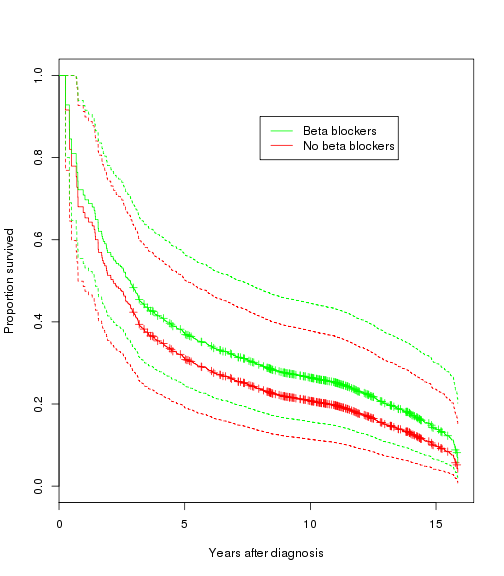### Testing for Proportional Hazards

Hypothesis test for proportional hazards:

# Note this is very conservative!
cox.zph(model)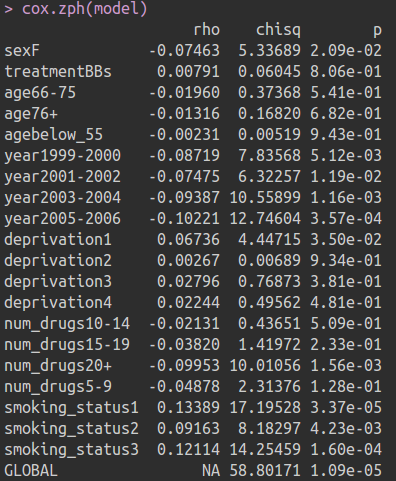### Testing for Proportional Hazards

It is often better to check the graphs for systematic trends:

a <- cox.zph(model)
par(mfrow = c(3, 1))
plot(a, main = "Sex")
plot(a, main = "diagnosis year")
plot(a, main = "Smoking status")### Accounting for non-proportional hazards

Include an interaction with time for the variables:

• This factors time out of the main effect
• Only use if it makes sense to have a linear interaction between the covariate and time (look at the graphs!)
mod2 <- coxph(S ~ sex + treatment + age +
year + deprivation +
num_drugs + year + year:stop + smoking_status,
data = colon)


Note you don't need to include stop as a main effect since it is already factored in S

### Accounting for non-proportional hazards

Alternatively, Stratify the data by that covariate

mod3 <- coxph(S ~ sex + treatment + age +
deprivation +
num_drugs + strata(year) +
smoking_status,
data = colon)

• Each strata has a different baseline hazard function but the remaining covariates are assumed to be constant
• Does not assume a linear relationship
• But you cannot examine the effects of the stratification variable
• though you can plot them …

### Visualising stratified survival curves

survfit plots a survival curve for each strata

plot(survfit(mod3), col = 1:5)
legend(11, 1,
legend = levels(colon\$year),
lty = 1,
col = 1:5,
title = "Diagnosis year")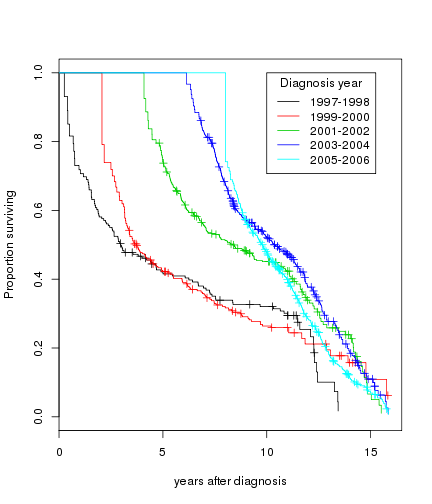### Non-independent observations

e.g. several plants in a family, patients in practices etc.

Deal with this by using cluster(variable):

mod3 <- coxph(S ~ sex + treatment + age +
deprivation +
cluster(practice) +
num_drugs + year +
smoking_status,
data = colon)

• Robust standard errors are produced taking into account the clusters
• Simpler than a full mixed-effects model